# Animation Pages 133 141 Function Function Definition Calling

• Slides: 20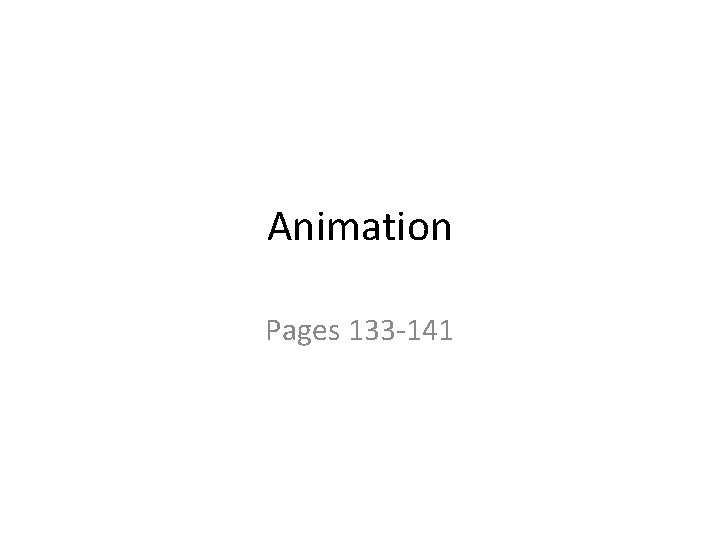Animation Pages 133 -141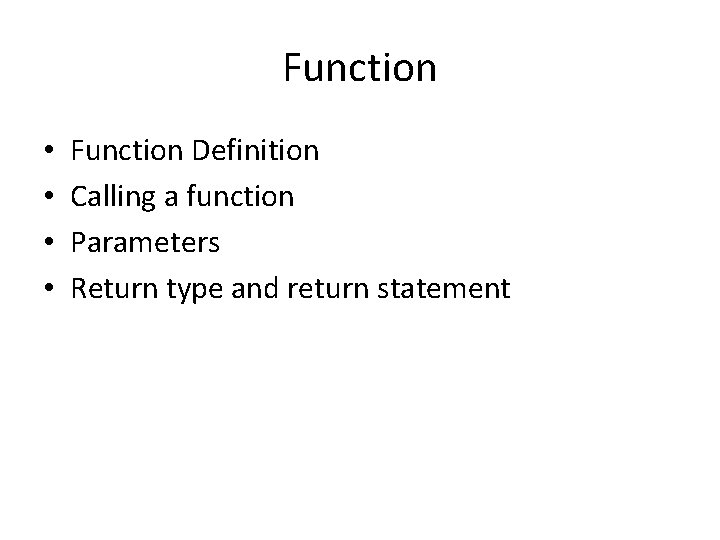Function • • Function Definition Calling a function Parameters Return type and return statementFunction: Intuitive Understanding X Function F F(x)Examples 10 X=10, y=4 Add 10 20 Multiply (x, y) Subtract 6 40 4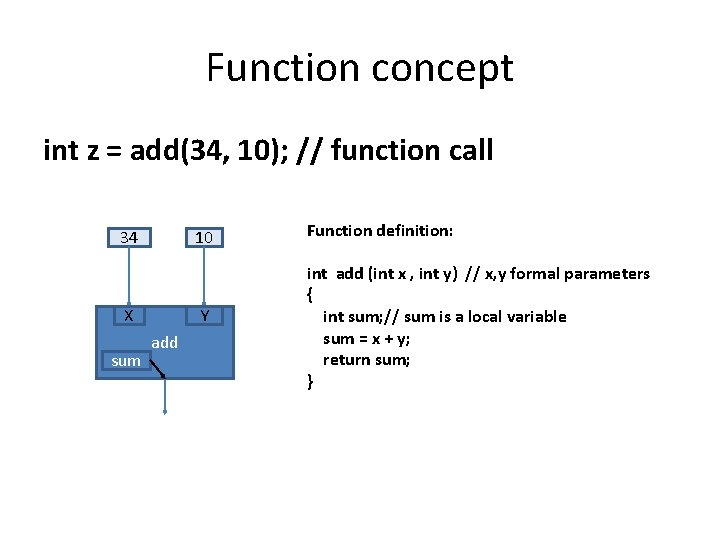Function concept int z = add(34, 10); // function call 34 10 X sum Y add Function definition: int add (int x , int y) // x, y formal parameters { int sum; // sum is a local variable sum = x + y; return sum; }translate Translate function moves the origin by the amount specified int x, y; x = 10; y = 10; void draw() { background(30, 50, 60); translate(x, y); rect(x, y, 70, 30); x++; if (x> width) x = 0; y++; if (y>height) y = 0; }Multiple objects • • • Consider a scenario with multiple objects Each with its own initial position Size Speed • How will you define these? How will control these? How will you detect collisions among these and take action?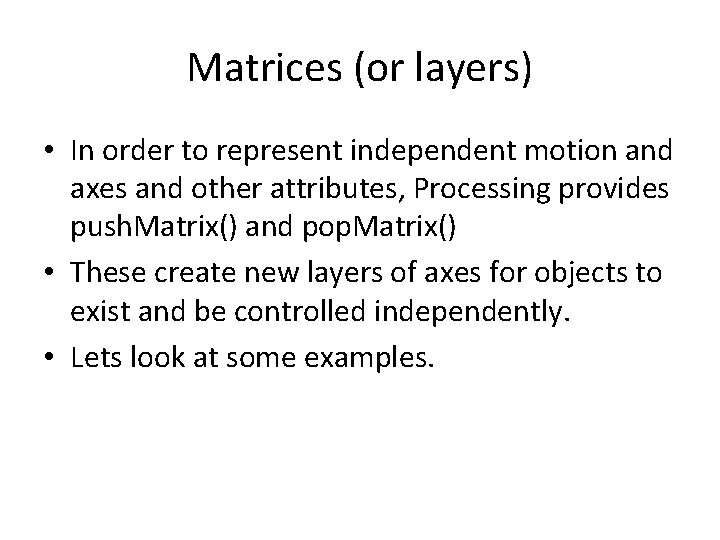Matrices (or layers) • In order to represent independent motion and axes and other attributes, Processing provides push. Matrix() and pop. Matrix() • These create new layers of axes for objects to exist and be controlled independently. • Lets look at some examples.Color by Numbers Pages 85 -93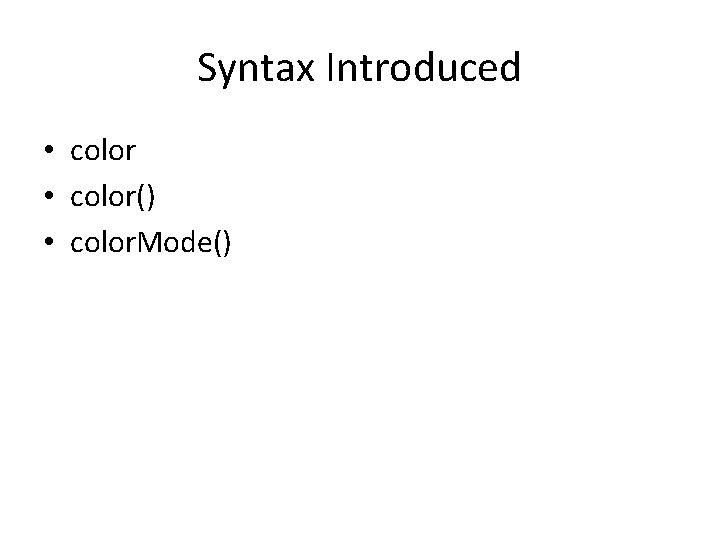Syntax Introduced • color() • color. Mode()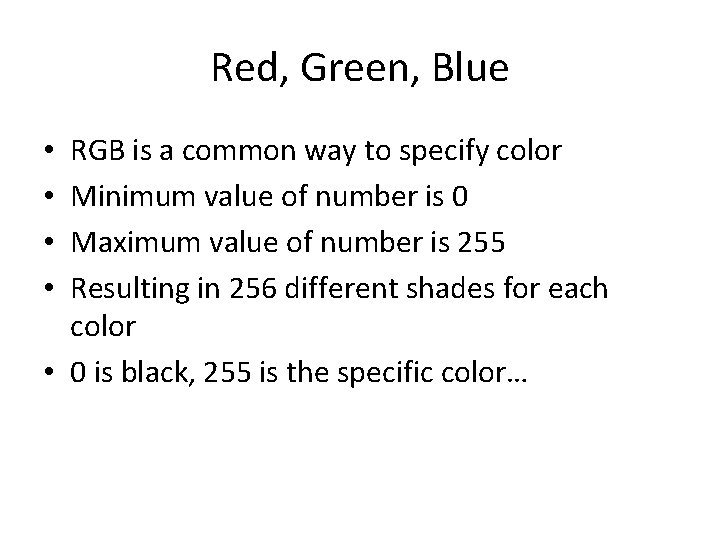Red, Green, Blue RGB is a common way to specify color Minimum value of number is 0 Maximum value of number is 255 Resulting in 256 different shades for each color • 0 is black, 255 is the specific color… • •Functions that take color as parameters background(r, g, b); fill(r, g, b, alpha) stroke(r, g, b); stroke(r, g, b, alpha) alpha is for opaque/transparency 0 – entirely transparent 255 – entirely opaque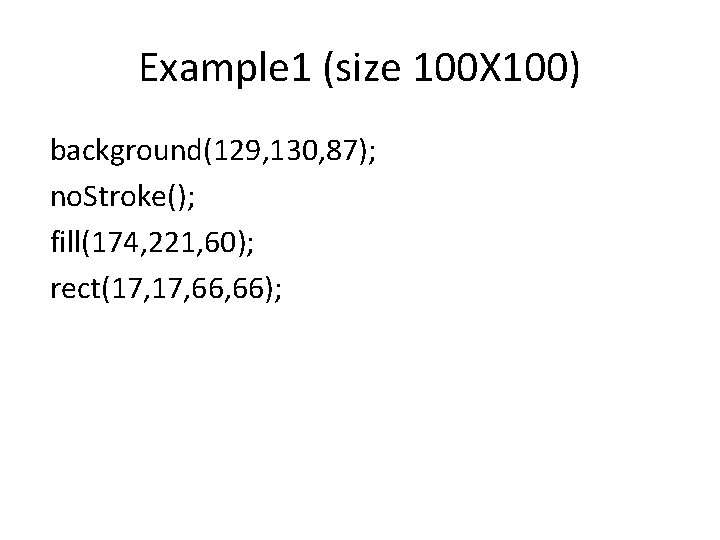Example 1 (size 100 X 100) background(129, 130, 87); no. Stroke(); fill(174, 221, 60); rect(17, 66, 66);Example 2 background(129, 130, 87); no. Fill(); stroke. Weight(4); stroke(174, 221, 60); rect(19, 62, 62);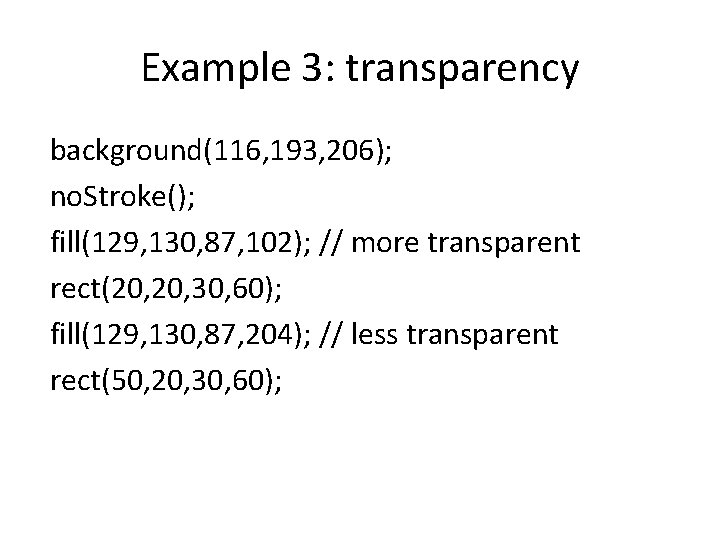Example 3: transparency background(116, 193, 206); no. Stroke(); fill(129, 130, 87, 102); // more transparent rect(20, 30, 60); fill(129, 130, 87, 204); // less transparent rect(50, 20, 30, 60);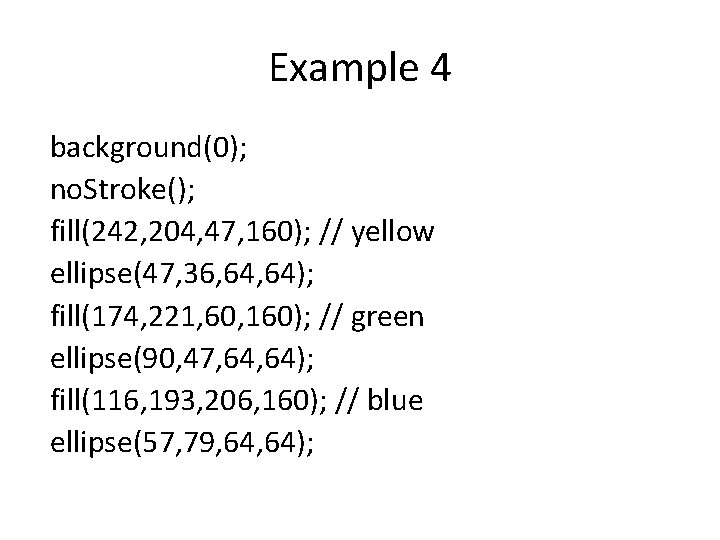Example 4 background(0); no. Stroke(); fill(242, 204, 47, 160); // yellow ellipse(47, 36, 64); fill(174, 221, 60, 160); // green ellipse(90, 47, 64); fill(116, 193, 206, 160); // blue ellipse(57, 79, 64);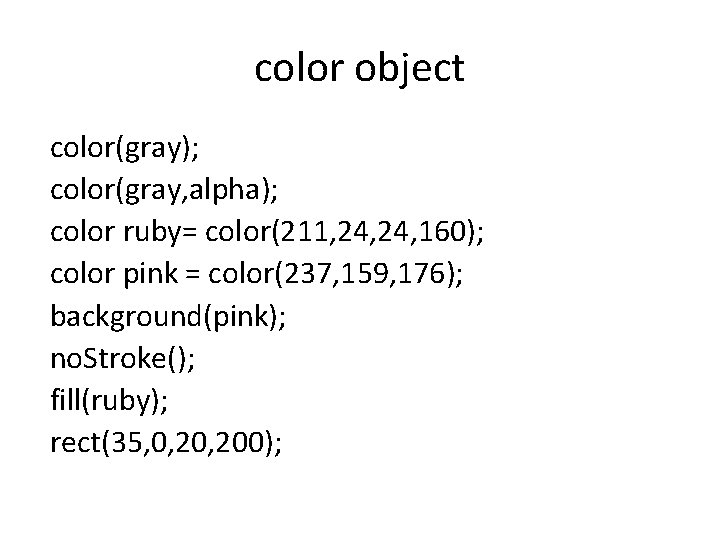color object color(gray); color(gray, alpha); color ruby= color(211, 24, 160); color pink = color(237, 159, 176); background(pink); no. Stroke(); fill(ruby); rect(35, 0, 200);Hue, Saturation, Brightness mode • Hue is the actual color. It is measured in angular degrees counter-clockwise starting and ending at red = 0 or 360 (so yellow = 60, green = 120, etc. ). • • Saturation is the purity of the color, measured in percent from (0) to (100). At 0% saturation, hue is meaningless. • • Brightness is measured in percent from black (0) to white (100). At 0% brightness, both hue and saturation are meaningless.Set a single pixel • Lets look at three program and review the code.Summary • We studied the usage of color and transparency • We also covered other color mode HSB (Hue, Saturation, Brightness) • We analyzed code using these attributed and some complex math.